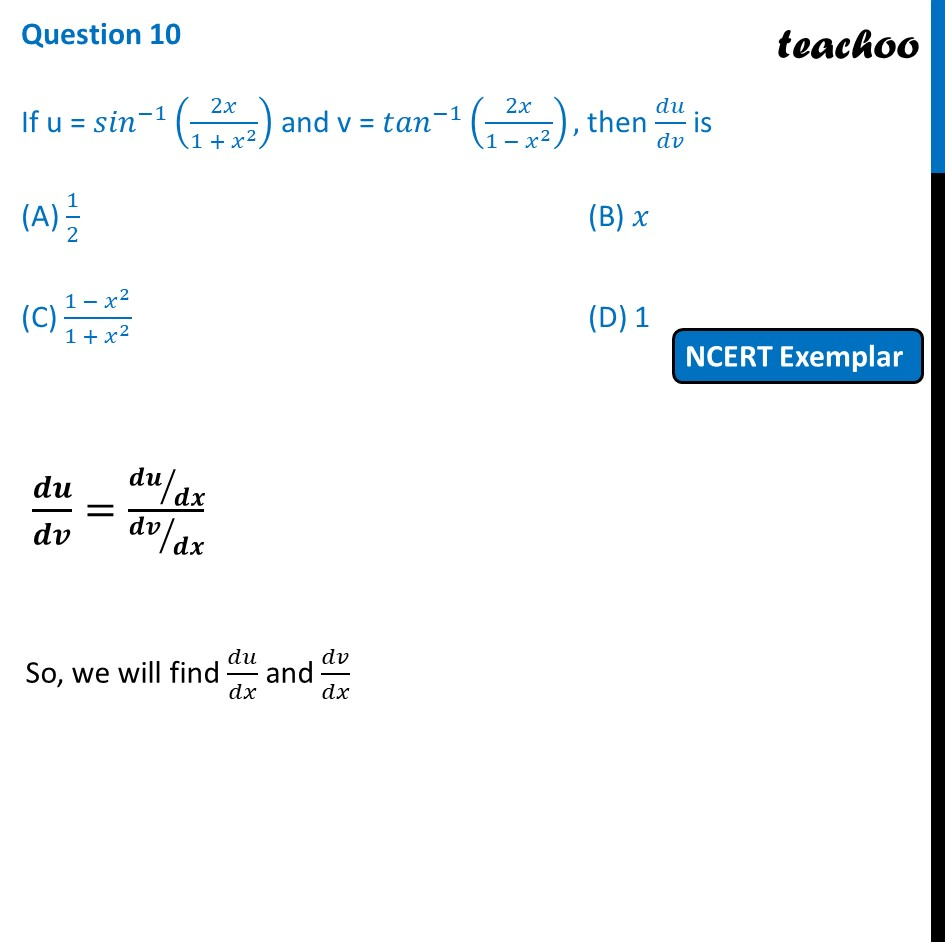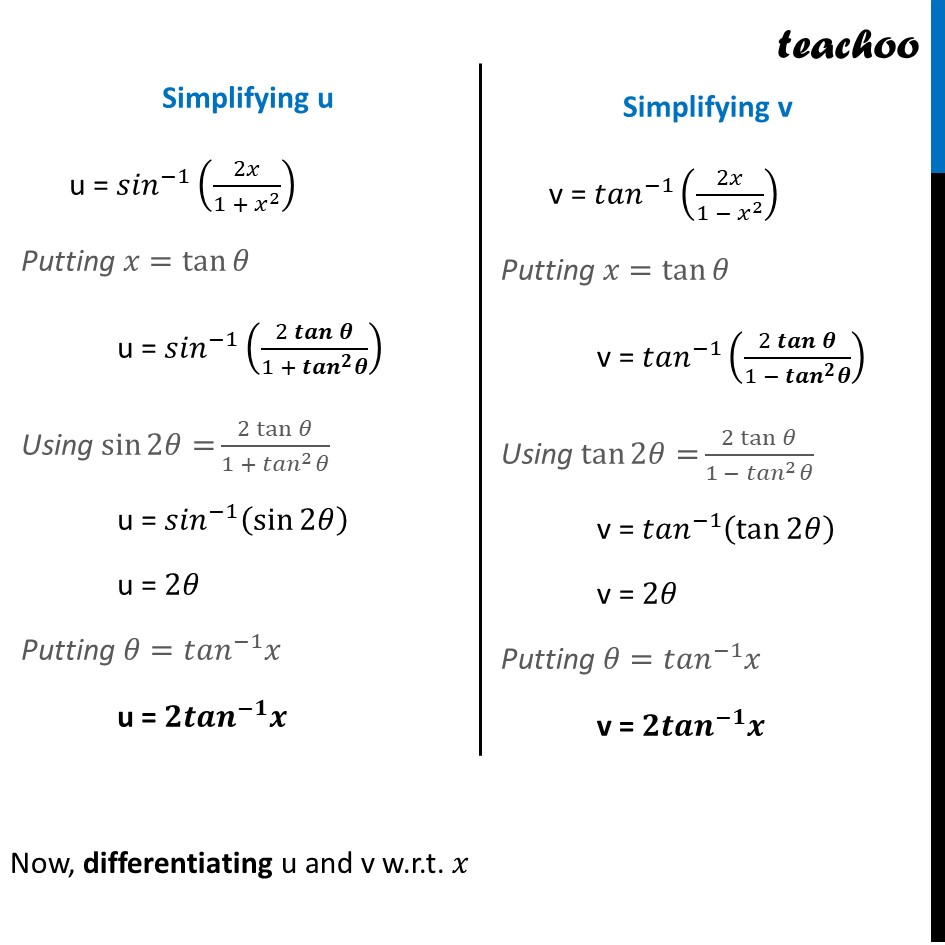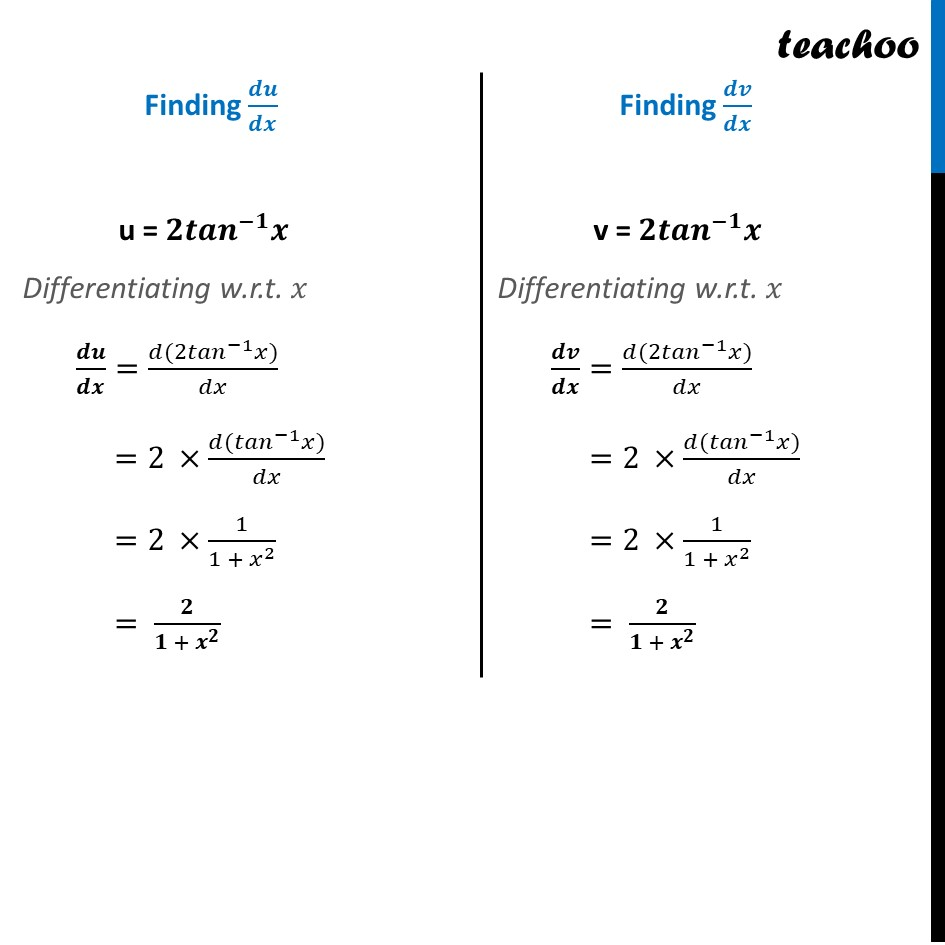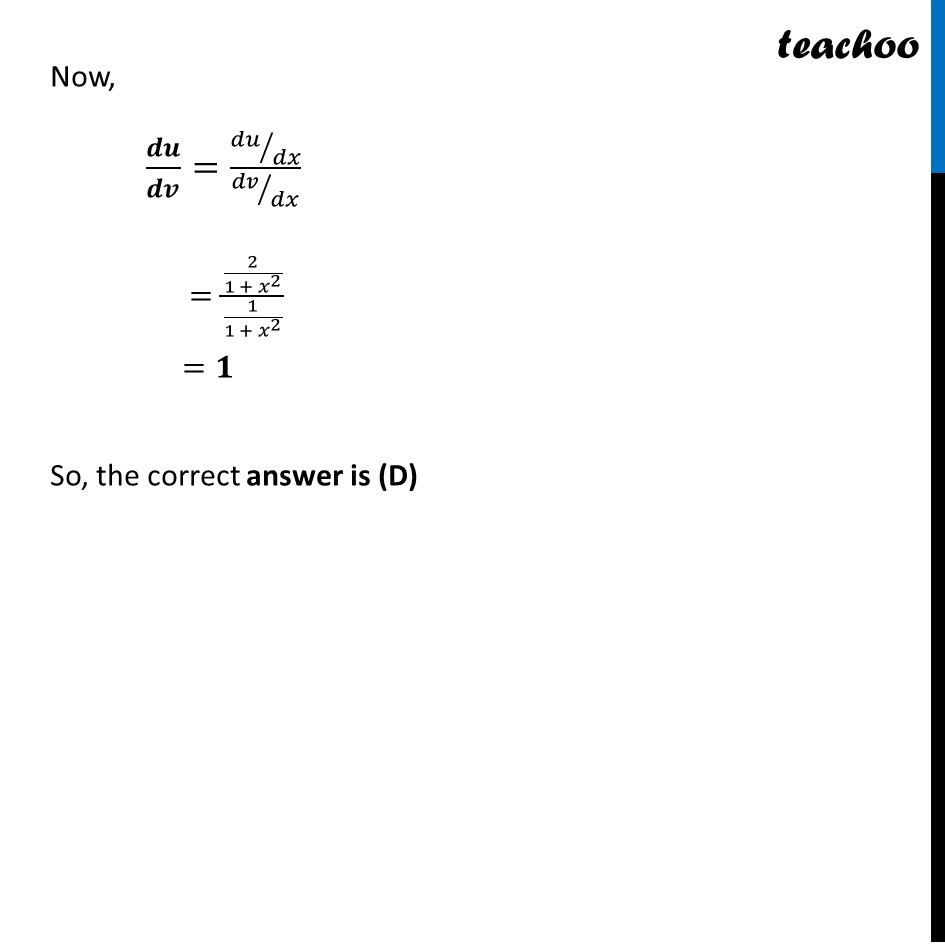NCERT Exemplar - MCQs

Chapter 5 Class 12 Continuity and Differentiability
Serial order wise

## (D) 1

This question is similar to Ex 5.3, 9 - Chapter 5 Class 12 - Continuity and DifferentiabilityGet live Maths 1-on-1 Classs - Class 6 to 12

### Transcript

Question 10 If u = 〖𝑠𝑖𝑛〗^(−1) (2𝑥/(1 + 𝑥^2 )) and v = 〖𝑡𝑎𝑛〗^(−1) (2𝑥/(1 − 𝑥^2 )), then 𝑑𝑢/𝑑𝑣 is (A) 1/2 (B) 𝑥 (C) (1 − 𝑥^2)/(1 + 𝑥^2 ) (D) 1 𝒅𝒖/𝒅𝒗=(𝒅𝒖⁄𝒅𝒙)/(𝒅𝒗⁄𝒅𝒙) So, we will find 𝑑𝑢/𝑑𝑥 and 𝑑𝑣/𝑑𝑥 Simplifying u u = 〖𝑠𝑖𝑛〗^(−1) (2𝑥/(1 + 𝑥^2 )) Putting 𝑥=tan⁡𝜃 u = 〖𝑠𝑖𝑛〗^(−1) ((2 𝒕𝒂𝒏⁡𝜽)/(1 + 〖𝒕𝒂𝒏〗^𝟐 𝜽)) Using sin⁡〖2𝜃=〗 (2 tan⁡𝜃)/(1 + 〖𝑡𝑎𝑛〗^2 𝜃) u = 〖𝑠𝑖𝑛〗^(−1) (sin⁡2𝜃 ) u = 2𝜃 Putting 𝜃=〖𝑡𝑎𝑛〗^(−1) 𝑥 u = 𝟐〖𝒕𝒂𝒏〗^(−𝟏) 𝒙 Simplifying v v = 〖𝑡𝑎𝑛〗^(−1) (2𝑥/(1 − 𝑥^2 )) Putting 𝑥=tan⁡𝜃 v = 〖𝑡𝑎𝑛〗^(−1) ((2 𝒕𝒂𝒏⁡𝜽)/(1 − 〖𝒕𝒂𝒏〗^𝟐 𝜽)) Using tan⁡〖2𝜃=〗 (2 tan⁡𝜃)/(1 − 〖𝑡𝑎𝑛〗^2 𝜃) v = 〖𝑡𝑎𝑛〗^(−1) (tan⁡2𝜃 ) v = 2𝜃 Putting 𝜃=〖𝑡𝑎𝑛〗^(−1) 𝑥 v = 𝟐〖𝒕𝒂𝒏〗^(−𝟏) 𝒙 Finding 𝒅𝒖/𝒅𝒙 u = 𝟐〖𝒕𝒂𝒏〗^(−𝟏) 𝒙 Differentiating w.r.t. 𝑥 𝒅𝒖/𝒅𝒙=(𝑑〖(2𝑡𝑎𝑛〗^(−1) 𝑥))/𝑑𝑥 =2 ×(𝑑〖(𝑡𝑎𝑛〗^(−1) 𝑥))/𝑑𝑥 =2 ×1/〖1 + 𝑥〗^2 = 𝟐/〖𝟏 + 𝒙〗^𝟐 Finding 𝒅𝒗/𝒅𝒙 v = 𝟐〖𝒕𝒂𝒏〗^(−𝟏) 𝒙 Differentiating w.r.t. 𝑥 𝒅𝒗/𝒅𝒙=(𝑑〖(2𝑡𝑎𝑛〗^(−1) 𝑥))/𝑑𝑥 =2 ×(𝑑〖(𝑡𝑎𝑛〗^(−1) 𝑥))/𝑑𝑥 =2 ×1/〖1 + 𝑥〗^2 = 𝟐/〖𝟏 + 𝒙〗^𝟐 Now, 𝒅𝒖/𝒅𝒗=(𝑑𝑢⁄𝑑𝑥)/(𝑑𝑣⁄𝑑𝑥) =(2/〖1 + 𝑥〗^2 )/(1/〖1 + 𝑥〗^2 ) =𝟏 So, the correct answer is (D)